Navigation: LaesieWorks homeThoughts homeThis pageSYMBOLSI and most of you often use these symbols:
0123456789
and
abcdefghijklmnopqrstuvwxyz

There are many others, like all Chinese symbols and the two digital.

Why counting decimal? 0123456789 (base 10 numeral system)
Just because we have 10 fingers? Or just because we are used to it?!
Is there a better option then 10?

What set of symbols is optimal for a human wanting to calculate simple calculations?

This is the most simple one: "Calculate A" (Base 1 numeral system)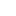=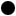= A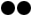= AA= AAA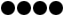= AAAA= AAAAA
And so on.
AAA + AA = AAAAA
AAAAA – AA = AAA
AAA : AA = A.(what to write here?!)
Three problems:
A) Writing large numbers, like "six thousand", is terrible.
AA) Not possible to write numbers smaller than A.
AAA) No symbol for "zero"

On step less simple is: "Calculate A-B" (Binary, or Base 2 numeral system)= A= B= BA= BB= BAA= BAB
And so on.

The conversion from decimal to A-B is some work, but A-B itself is very easy. It may seem hard, but that's if you're not familiar with it.
The first symbol is on the RIGHT side, were A = zero, and B = one.
The next symbols:
A is always zero, and
B always is two times the value of the previous B. (if there would be one).

BBBBBBBBBB = 512, 256, 128, 64, 32, 16, 8, 4, 2, 1 (all together 1023)
AAAAAAAAAA = 0, 0, 0, 0, 0, 0, 0, 0, 0, 0, (all together 0)

BABA = 8, 0, 2, 0 (all together 10)
ABBA = 0, 4, 2, 0 (all together 6)

 A A –– + A (0 + 0 = 0) A B –– + B (0 + 1 = 1) B A –– + B (1 + 0 = 1) B B –– + BA (1 + 1 = 2)

Simple, not?!

BBA
AAB
–––– +
BBB

(this was 8 + 8 = 16)

BAA
BAA
–––– +
BAAA

(this was 8 + 8 = 16)

BAAABBA (64+0+0+0+4+2+0 = 70)
BAABBBB (64+0+0+8+4+2+1 = 79)
–––––––––– +
BAABABAB (128+0+0+16+0+4+0+1 = 149)

In steps, from right to left:
A + B = B. Note a B.
B + B = BA. Note an A and remember B.
B + B + B = BB. Note a B and remember B.
A + B + B = BA. Note an A and remember B.
A + A + B = B. Note a B.
A + A = A. Note an A.
B + B = BA. Note BA.

When if "calculating A-B" is the only tool you use to calculate, then you'll only need to remember a few things:

A + A = A
A + B = B

B + A = B
B + B = BA
and
B + B + B = BB

A - A = A
A - B = -B

B - A = B
B - B = A
B - B - B = -B

A
x A = A
A
x B = A
B x A = A
B
x B = B
and
BA x BA = BAA
BAA
x BAA = BAAAA
BAAA
x BAAA = BAAAAAA
(and so on)

Reasons to use "Calculate A-B"
- Easy to learn
- More advanced then "Calculate A"
- Easy to upgrade to "Calculate A-D"
- Digital computers use "Calculate A-B"
Reasons to NOT use "Calculate A-B"
- "Please give me BABB of these" (eleven). There are so few symbols, too few to be practical for everyday use.

Which brings me to the next topic:
How many dots can YOU recognize at once?
(this will be different from person to person)
If there are 5 dots, do you see 5 dots, or 4 + 1?
Or maybe 3 + 2, or 2 + 2 + 1, or 1 + 1 + 1 + 1 + 1 + 1?
A baby starts with recognizing 1 thing at a time
I'm not really into math and think I can recognize 4 dots at once, but I'm sure some will be able to recognize larg numbers of dots at once.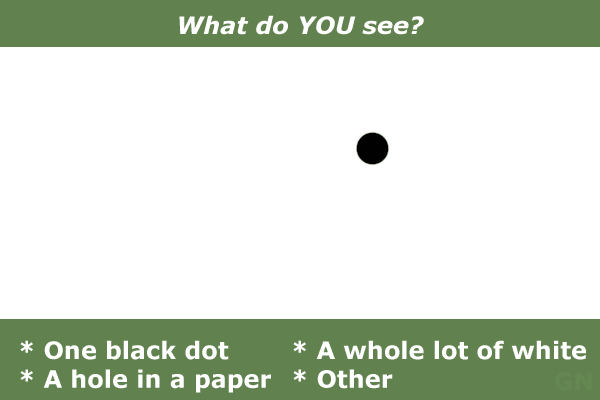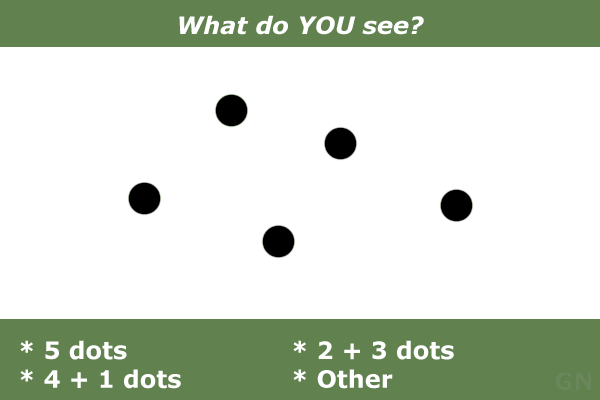Check this out:= 0 = zero= 1 = one= 2 = two= 3 = three= 4 = four= 5 = five= 6 = six= 7 = seven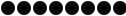= 8 = eight= 9 = nine= 10 = ten= 11 = eleven (should be "ten - one")= 12 = twelve (should be "ten - two")= 13 = thir - teen (should be "ten - three")= 14 = four - teen (should be "ten - four")= 15 = fif - teen (should be "ten - five")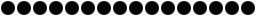= 16 = six - teen (should be "ten - six")
17 = seven - teen (should be "ten - seven")
18 = eigh - teen (should be "ten - eight")
19 = nine - teen (should be "ten - nine")
20 = twenty
21 = twenty - one
22 = twenty - two
23 = twenty - three
24 = twenty - four
25 = twenty - five
and so on, with only some unique names:
30 = thirty
40 = forty
50 = fifty
60 = sixty
70 = seventy
80 = eighty
90 = ninety
100 = one hundred
1000 = one thousand
10000 = ten thousand (not unique)
100000 = one hundred thousand (not unique)
1000000 = one million
Many other names, there are.
Point of this row of numbers and names is to see that we have names for:
- Zero to Twelve
- Multiples of 10
- Multiples of 100 x 10

But why are there names from Zero to Twelve? For some historical cause, or is 12 more efficient then 10?

Base 1
1 : 1 = 1

Base 2
2 : 1 = 2
2 : 2 = 1

Base 3
3 : 1 = 3
3 : 2 = 1 1/2
3 : 3 = 1

Base 4
4 : 1 = 3
4 : 2 = 2
4 : 3 = 1 1/4
4 : 4 = 1

Base 5

Base 6

Base 7

Base 8
8 : 2 = 4
8 : 3 = 2 2/3
8 : 4 = 2

Base 9
9 : 2 = 4 1/2 (or 4 4/8)
9 : 3 = 3
9 : 4 = 2 1/4 (or 2 2/8)
9 : 5 = 1 4/5
9 : 6 = 1 1/2 (or 2 4/8)
9 : 7 = 1 2/7
9 : 8 = 1 1/8

Base 10 (Decimal)
10 : 2 = 5
10 : 3 = 3 1/3 (or 3 2/6)
10 : 4 = 2 1/2 (or 2 3/6)
10 : 5 = 2

Base 11

Base 12
12 : 2 = 6
12 : 3 = 4
12 : 4 = 3
12 : 5 = 2 2/5 (or 2 4/10)
12 : 6 = 2

Base 13

Base 14

Base 15

Base 16
16 : 2 = 8
16 : 3 = 5 1/3
16 : 4 = 4
16 : 5 = 3 1/2
16 : 6 = 2 2/3
16 : 7 = 2 2/7
16 : 8 = 2

You may now forget this (and some other tables too):
 1x1 = 1 2x1 = 2 3x1 = 3 4x1 = 4 5x1 = 5 6x1 = 6 7x1 = 7 8x1 = 8 9x1 = 9 10x1 = 10 1x2 = 2 2x2 = 4 3x2 = 6 4x2 = 8 5x2 = 10 6x2 = 12 7x2 = 14 8x2 = 16 9x2 = 18 10x2 = 20 1x3 = 3 2x3 = 6 3x3 = 9 4x3 = 12 5x3 = 15 6x3 = 18 7x3 = 21 8x3 = 24 9x3 = 27 10x3 = 30 1x4 = 4 2x4 = 8 3x4 = 12 4x4 = 16 5x4 = 20 6x4 = 24 7x4 = 28 8x4 = 32 9x4 = 36 10x4 = 40 1x5 = 5 2x5 = 10 3x5 = 15 4x5 = 20 5x5 = 25 6x5 = 30 7x5 = 35 8x5 = 40 9x5 = 45 10x5 = 50 1x6 = 6 2x6 = 12 3x6 = 18 4x6 = 24 5x6 = 30 6x6 = 36 7x6 = 42 8x6 = 48 9x6 = 54 10x6 = 60 1x7 = 7 2x7 = 14 3x7 = 21 4x7 = 28 5x7 = 35 6x7 = 42 7x7 = 49 8x7 = 56 9x7 = 63 10x7 = 70 1x8 = 8 2x8 = 16 3x8 = 24 4x8 = 32 5x8 = 40 6x8 = 48 7x8 = 56 8x8 = 64 9x8 = 72 10x8 = 80 1x9 = 9 2x9 = 18 3x9 = 27 4x9 = 36 5x9 = 45 6x9 = 54 7x9 = 63 8x9 = 72 9x9 = 81 10x9 = 90 1x10 = 10 2x10 = 20 3x10 = 30 4x10 = 40 5x10 = 50 6x10 = 60 7x10 = 70 8x10 = 80 9x10 = 90 10x10 = 100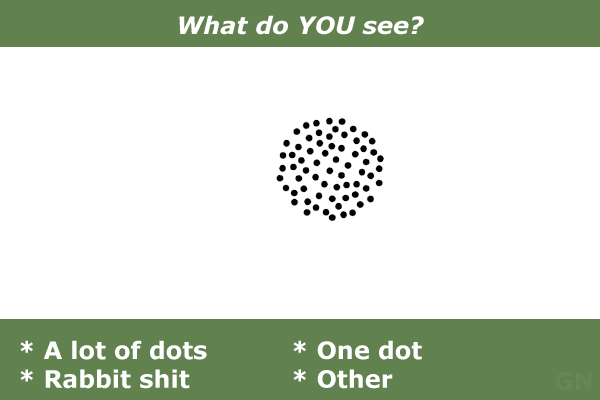Giesbert Nijhuis